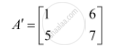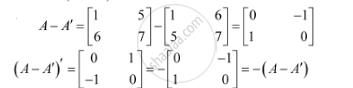Share

# For the Matrix a = [(1,5),(6,7)] Verify That (A - A') Is a Symmetric Matrix - CBSE (Science) Class 12 - Mathematics

ConceptSymmetric and Skew Symmetric Matrices

#### Question

For the matrix A = [(1,5),(6,7)] verify that (A - A') is a skew symmetric matrix

#### SolutionHence, (A - A') is a skew-symmetric matrix.

Is there an error in this question or solution?

#### Video TutorialsVIEW ALL 

Solution For the Matrix a = [(1,5),(6,7)] Verify That (A - A') Is a Symmetric Matrix Concept: Symmetric and Skew Symmetric Matrices.
S